Algebra: Solving Linear Equations – Part 1: The BasicsAlgebra: Solving Linear Equations – Part 1: The Basics

[NOTE: This video is only the first 18 minutes of the full lesson, please visit my website to purchase complete version] Lesson consists of providing you with a Self-Tutorial on how to solve linear equations. Tutor discusses what is an Equation, Solution, Solution Set, Equivalent Equations, Identity, Contradictions, Conditional Equations, Linear Equations, the Standard Form of a Linear Equation. I show you how to actually SOLVE linear equations going step-by-step. I don’t skip steps. I review some of the properties of Equality here too. I remind you to check the work done and how to solve linear equations using a calculator. At the end, you will know how to easily solve equations that look like:

2[y – (4y – 1)] = 5 – 9y

No word problems are done here. This is shown in DETAIL in Part 2 of the Lesson.

algebra tutors,math,tutor,tutoring,tutorial,arithmetic,algebra,calculus,geometry,school,college,test,prep,SAT,ACT,AIMS,study,tips,graph,vmtalgsolvlineq1

algebratutors.org

21 Responses to “Algebra: Solving Linear Equations – Part 1: The Basics”

1.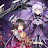nina rosee says:

i love math ><

2.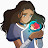amaramation says:

I thought the music was from ROUND THE TWIST so i was about to go

HAVE YOU EVER

EVER FELT LIKE THIS

3.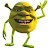Jacob says:

I should probably listen more in my math class

4.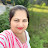Counseling Expert view says:

3 year ago, gulshan was 5 years older than his sister divya was than if he is now twice as old as she is find the present ages plz solve it

5.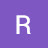Ramandeep Chandi says:

how does 9 equal 9 I'm so confused and I have a test due help

6.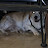David Gonzalez says:

This may sound dume but.how dose 9=9

7.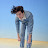xoxo hello says:

I just finished my math test and probably will get an F lol and last night i kept on searching for linear equation tutorials and they make it seem so hard. NOW I KNOW HOW THIS WORKS AND I MY MATH TEST IS FINISHED. OKAY

8.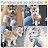Stella Loves Dogs says:

It helped me a lot👌🏻! Thanks for this tutor video😁!

9.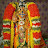Sowjanya Chelikani says:

10.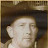EMC2 says:

Luis, … At the beginning you verbally state; … 2 + 3 = 5, and the screen shows 2+2=4 & 1+1=3. ….. I don't know if I am the first to notice this, … but, there you are, you made a boo boo.

11.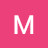Mr.SlimGold says:

Why can't I hear any audio speaking through my right earbud but I hear it perfectly through my left

12.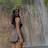Karl Heinz Tala says:

i definitely understand everything now! my teacher in math doesn't make sense in teaching (back in sophomore) and now that I'm in 9th grade I have no Idea when my teacher ask if is it a linear equation or whatsoever questions. now, I finally understand it!

13.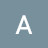Ams S says:

Very good video ,helped me alot with my study.Also I love the 80s sounding intro at the beginning!

14.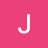Jakeylla Harrison says:

linear equations are complicated so thank. you you just made it a little bit more sense

15.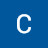Charley Mao says:

16.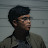nathaniel ciriaco says:

and you are like my teacher. 🙂

17.nathaniel ciriaco says:

hi video math tutor thanks for the math lesson i need it in high school.

18.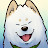duplicarto says:

Hold on, did that 1 + 1 equation just have number 3 for an answer?

19.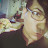sid 97 says:

actually i have a doubt in example 4 – you said 1/2x=3y a linear equation because both the variables x and y are present as numerators but in the case of  1/2x=3/y you said it is nonlinear how come you can use reciprocal method to keep them both as numeraotors as 1/2x=3/y is same as y=6x   please help me on this

20.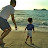Rexel Agapay says:

Thanks! Your video has helped me a lot 🙂

21.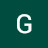Greg Rorrer says:

Where can I get your notes that I can use to follow along with you?